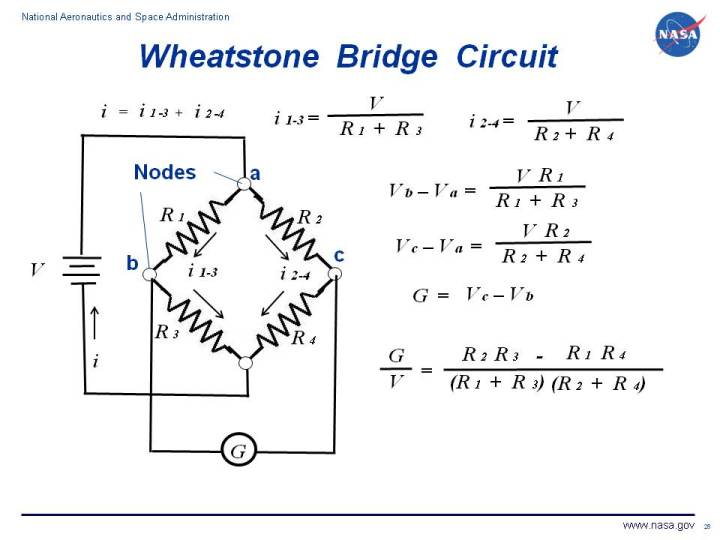+ Text Only Site
+ Non-Flash Version
+ Contact GlennElectrical circuits are used throughout aerospace engineering, from flight control systems, to cockpit instrumentation, to engine control systems, to wind tunnel instrumentation and operation. The most basic circuit involves a single resistor and a source of electric potential or voltage. Electrons flow through the circuit producing a current of electricity. The resistance, voltage, and current are related to one another by Ohm's law. There is usually more than one resistor used in a practical circuit. In the analysis of circuits with multiple resistors, we must determine if the resistors are subject to the some voltage or to the same current. Multiple resistors in a parallel circuit are subjected to the same voltage. Multiple resistors in a series circuit are subjected to the same current. On this page we discuss an the Wheatstone bridge circuit which is an important circuit that is used in wind tunnel instrumentation If we denote resistance by R, current by i, and voltage by V, then Ohm's law states that for each resistor in the circuit: V = i R i = V / R On the figure, we show a circuit consisting of a power source and four resistors connected in a square. The resistors are connected to each other at nodes which are labeled a through c. The circuit contains a potentiometer, labeled G, which detects the voltage difference between nodes c and b. The value from the potentiometer is displayed in the control room. If we consider each resistor separately, each resistor has its own current (i1, i2, i3, and i4), resistance (R1, R2, R3 , and R4), and voltage (V1, V2, V3, and V4), which are related to each other through Ohm's law. In practice, the resistors would actually be the resistance provided by a strain gage in a wind tunnel force balance system. Resistors R1 and R3 are connected in series through node b. Therefore the same current flows through R1 and R3. i(1-3) = i1 = i3 and the value of i(1-3) can be determined from Ohm's law: i(1-3) = V / (R1 + R3) Similarly, resistors R2 and R4 are connected in series and the same current i(2-4) flows through these resistors. The current is given by: i(2-4) = V / (R2 + R4) The change in voltage from nodes a to node b is given by: Vb - Va = i(1-3) R1 = V R1 / (R1 + R3) Similarly, the voltage change from node a to node c is given by: Vc - Va = i(2-4) R2 = V R2 / (R2 + R4) The potentiometer G measures the difference in voltage between nodes b and c. G = Vc - Vb = (Vc - Va)- (Vb - Va) G = V [ {R2 / (R2 + R4)} - {R1 / (R1 + R3)} ] G / V = [(R2 R3) - (R1 R4)] / [(R1 + R3) (R2 + R4)] This final equation explains how a Wheatstone bridge circuit can be used to eliminate temperature bias when using a strain gage to determine forces on a wind tunnel model. Two strain gages are connected to the model, and the output from the gages are put into a Wheatstone bridge as R1 and R2. Equal "ballast" resistors are placed in R3 and R4. If the gage is subjected to an increase in temperature, the resistance in both R1 and R2 increase by the same amount. But because the potentiometer measures the difference in resistance between R1 and R2, the reading stays the same. Navigation ..Beginner's Guide Home Page+ Inspector General Hotline + Equal Employment Opportunity Data Posted Pursuant to the No Fear Act + Budgets, Strategic Plans and Accountability Reports + Freedom of Information Act + The President's Management Agenda + NASA Privacy Statement, Disclaimer, and Accessibility CertificationEditor: Nancy Hall NASA Official: Nancy Hall Last Updated: May 05 2015 + Contact Glenn